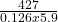## a lead block drops its temperature by 5.90 degrees celsius when 427 J of heat are removed from it. what is the mass of the block?(unit=kg) I

Question

a lead block drops its temperature by 5.90 degrees celsius when 427 J of heat are removed from it. what is the mass of the block?(unit=kg) IM GIVING 30 POINTS FOR THE CORRECT ANSWER

in progress 0
3 weeks 2021-09-03T17:48:42+00:00 2 Answers 0 views 0

577g

Explanation:

Given parameters:

Temperature change = 5.9°C

Amount of heat lost = 427J

Unknown:

Mass of the block = ?

Solution:

The heat capacity of a body is the amount of heat required to change the temperature of that body by 1°C.

H =  m c Ф

H is the heat capacity

m  is the mass of the block

c is the specific heat capacity

Ф is the temperature change

Specific heat capacity of lead is 0.126J/g°C

m = H / m Ф

m == 577g

Mass of the lead block is 577g